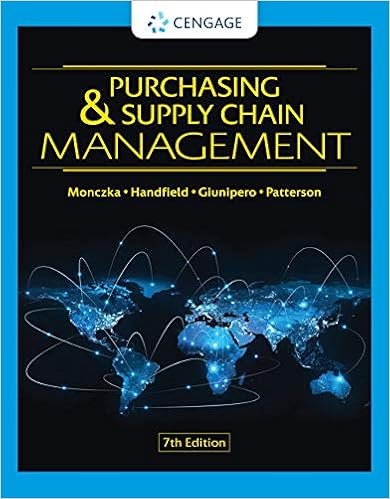# conceptflashcards3 - 1 What is cost assignment 2 What is a...

• Notes
• Paress
• 9

This preview shows page 1 - 2 out of 9 pages.

##### We have textbook solutions for you!
The document you are viewing contains questions related to this textbook.The document you are viewing contains questions related to this textbook.
Chapter 19 / Exercise 11
Handfield/MonczkaExpert Verified
1 What is cost assignment?2 What is a cost object?3 What is a direct cost of a cost object?4 What is an indirect cost of a cost object?5 What are the four reasons we allocate costs?6 What criteria should be used in allocating cost?7 Is any criteria generally more important than the others?8 What is a cost pool?9 What is an allocation base?10 To allocate indirect cost to a cost object, what must we know?11 What do we get if we divide cost pool by the total allocation base?1213 When might a company use a job cost system?14 In a job cost system what is the typical cost pool?15 In a job cost system what is typically the cost object?16 How do we trace direct costs in a job cost system?17 What is a subsidiary ledger account?18 What is a control account?19 What is a job cost sheet?20 What are examples of types of companies that might use job cost system?21 Describe a normal costing system?22 Describe an actual costing system?23 What are the trade-offs between using a normal and actual costing system?24 How do manufacturing costs flow to the income statement in a job cost system?25 Describe an over-costed product:26 What problems can occur if products are over-costed?27 Describe an under-costed product:28 What problems can occur if products are under-costed?293031 What are three signs that a company might need a refined cost system?32 What are three guidelines for refining a cost system?333435 Describe an activity based costing system.36 What are output unit-level cost?37 What is a batch-level cost?38 What is a product-sustaining cost?39 What is a facility sustaining cost?40 What non-manufacturing costs might be considered when determining product costs?4142 What is a process costing system?43 What is true about a manufacturing plant if process costing to be used?44 Why do we assign costs to units in each department?45 What are conversion costs?46 What is a physical unit? 47 What are the two method of assigning costs to units that we discussed?48 What is the formula for estimating cost per eu using weighted average?49 What is the formula for estimating cost per eu using FIFO?50 What is the advantage of FIFO over weighted average methods51 What explains the difference in cost per equivalent unit between the two methods?52 What are the possible cost pools to be allocated in a process costing system?53 Are direct materials added in every department?54 What is the allocation base used to assign costs in a process costing system?5556 What are transferred-in costs?57 Why should we separate transferred in cost from material cost?58 What are joint costs?59 What is the split-off point?60 What are joint products?61 What is a main product?62 What is a by-product? 63 What is a separable or additional processing costs?64 What are reasons for allocating joint costs?65 What are some types of products that might require joint cost allocation?66 What is the cost pool in joint cost allocation?67 What are the cost objects in joint cost allocation?68 What are four methods to allocate joint costs?
##### We have textbook solutions for you!
The document you are viewing contains questions related to this textbook.The document you are viewing contains questions related to this textbook.
Chapter 19 / Exercise 11Expert Verified
•••